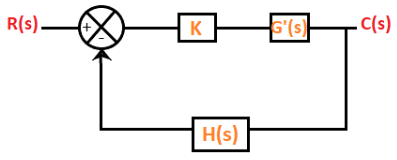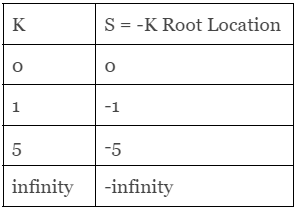Basic Concepts of Root Locus

# Basic Concepts of Root Locus - Notes | Study Control Systems - Electrical Engineering (EE)

 Table of contentsIntroduction1 Crore+ students have signed up on EduRev. Have you?

Introduction

The root locus method was introduced by W.R Evans in 1948. Root locus is a graphical method in which the movement of poles in the s-plane can be located when a specific parameter is varied from 0 to infinity. The parameter assumed to be varied is generally the gain of the system.
Consider the below closed loop system.The equation of a closed loop system is given by:
1 + G(s)H(s) = 0
Where,
G(s) is the gain of the transfer function
H(s) is the feedback gain
In the case of root locus, the gain K is also assumed as part of the closed-loop system. K is known as system gain or the gain in the forward path. The characteristic equation after including the forward gain can be represented as:
1 + KG'(s)H(s) = 0
Where,
G(s) = KG'(s)
When the system includes the variable parameter K, the roots of the closed loop system are now dependent on the values of 'K.'
The value of 'K' variable can vary in two cases, as shown below:

1. -∞ to +∞
2. 0 to +∞

In the first case, for every different value (integer or decimal) of K, we will get separate set of locations of the roots. If all such locations are joined, the resulting plot is defined as the root locus. We can also define root locus as the locus of the closed loop poles obtained when the system gain 'K' is varied from -infinity to infinity. When the K varies from zero to infinity, the plot is called the direct root locus. If the system gain 'K' varies from -infinity to zero, the plot thus obtained is known as inverse root locus. The gain K is generally assumed from zero to infinity unless specially stated.

Let's consider an example.
Example: Obtain the root locus of the unity feedback system with G(s) = K/s.

As given, it is a unity feedback system. It means that H(s) = 1.
We know,
1 + G(s)H(s) = 0
1 + K/s = 0
S + K = 0
The roots of the above equation are located at s = -K.

As per the condition, the system gain K varies from zero to infinity unless states. Thus, we will obtain the root locus by joining all such locations when K varies from zero to infinity. The values of the roots of the given equation at different values of K are given in the below table:The plot of the root locus for the above values of K is shown below:

Uses of Root Locus

In addition in determining the stability of the system, root locus also helps to determine:

• Damping ratio: The damping ratio is a dimensionless unit that describes how the system decay affects the oscillations of the system.
• Natural frequency: It is represented by wn. The value of the system gain K at the location of poles helps in computing the natural frequency and the damping ratio of the system.
• P, PI, and PID controllers: P (proportional), PI (Proportional Integral), and PID (Proportional Integral Derivative) controllers can be designed with the help of root locus technique. Here, the input of the system to be controlled is made proportional to the system gain K.
• Lag and lead compensators: The compensators are the additional components in the system added to compensate for deficient performance. The phase lead compensator helps to shift the root locus towards the left in the complex s-plane, and it further increases the system's stability. Similarly, lag and lead compensators can be designed in various ways with the help of the root locus.

The advantages of root locus are as follows:

• We can analyze the absolute stability of the system with the help of a root locus plot.
• Using the magnitude and angle conditions, we can find the limiting value of the system gain K for any point on the root locus.
• Enhances system designing with better accuracy.
• It helps in analyzing the stability of the system with time delay.
• Root locus plots help us determine the gain margin, relative stability, phase margin, and the system's settling time.
• The root locus technique is easy to implement as compared to other techniques in the control system.
• It helps in analyzing the performance of the control system.
The document Basic Concepts of Root Locus - Notes | Study Control Systems - Electrical Engineering (EE) is a part of the Electrical Engineering (EE) Course Control Systems.
All you need of Electrical Engineering (EE) at this link: Electrical Engineering (EE)

## Control Systems

54 docs|45 tests
 Use Code STAYHOME200 and get INR 200 additional OFF

## Control Systems

54 docs|45 tests

Track your progress, build streaks, highlight & save important lessons and more!

,

,

,

,

,

,

,

,

,

,

,

,

,

,

,

,

,

,

,

,

,

;